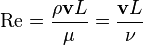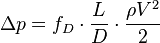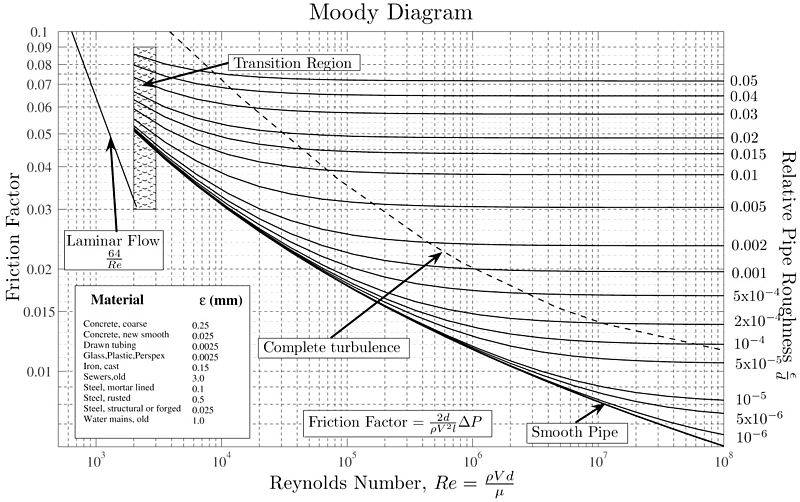# [Fluid Mechanics] Water Flow in a Hose

## Homework Statement

Given:
Water flows through a 15.24m long, 1.6cm diameter hose.

pressure at the outdoor tap = 275.8kPa

volumetric flowrate, Q = 7.5e-4 m^3/s (i.e. 7.5L for 10 seconds)

viscosity of water, μ = 0.001 Pa*s
density of water, ρ = 1000 kg/m^3

Look for: the roughness of the hose

## Homework Equations

Renolds number:Darcy equation:Moody chart:## The Attempt at a Solution

I have calculated Renolds number = 7958 ==> turbulent flow

I used Darcy equation and found out the friction factor, f, is great than 1.
I am confused and stuck here.
I must have used the wrong formula or missed some important steps.

Please help me by providing some advice, double checking my work or giving some steps would be greatly appreciated!

## Answers and Replies

Chestermiller
Mentor
Show us your work.

volumetric flowrate = Q = 7.5e-4 m^3/s
D = diameter = 0.016m
velocity = V =volumetric flow rate/cross sectional area = Q/(pi*D^2*0.25) = 0.497 m/s

density = ρ = 1000kg/m^3
viscosity = μ = 0.001 Pa*s
Renolds number = Re = (density*velocity*diameter)/viscosity = 7958 > 2100 ==> turbulent flow

L = length of the hose = 15.24m
pressure at outdoor tap = ΔP = 275.8kPa
friction factor = f = (2*ΔP*D) / (L*ρ*V^2) = 2.34 ==> doesn't make sense and I'm stuck here.

Chestermiller
Mentor
Check your velocity calculation. I get 3.73 m/s.

Thank you very much, Chestermiller!

you are correct, V = 3.73 m/s,
problem solved.